## Section3.5Random variables

###### Random Variables video

Two books are assigned for a statistics class: a textbook and its corresponding study guide. The university bookstore determined 20% of enrolled students do not buy either book, 55% buy the textbook only, and 25% buy both books, and these percentages are relatively constant from one term to another. If there are 100 students enrolled, how many books should the bookstore expect to sell to this class?

Solution

Around 20 students will not buy either book (0 books total), about 55 will buy one book (55 books total), and approximately 25 will buy two books (totaling 50 books for these 25 students). The bookstore should expect to sell about 105 books for this class.

Would you be surprised if the bookstore sold slightly more or less than 105 books? 1 If they sell a little more or a little less, this should not be a surprise. Hopefully Chapter 2 helped make clear that there is natural variability in observed data. For example, if we would flip a coin 100 times, it will not usually come up heads exactly half the time, but it will probably be close.

The textbook costs $137 and the study guide$33. How much revenue should the bookstore expect from this class of 100 students?

Solution

\begin{gather*} 137 \times 55 = 7,535 \end{gather*}

The roughly 25 students who buy both the textbook and the study guide would pay a total of

\begin{gather*} (137 + 33) \times 25 = 170 \times 25 = 4,250 \end{gather*}

Thus, the bookstore should expect to generate about $7,535 + 4,250 = 11,785$ from these 100 students for this one class. However, there might be some sampling variability so the actual amount may differ by a little bit.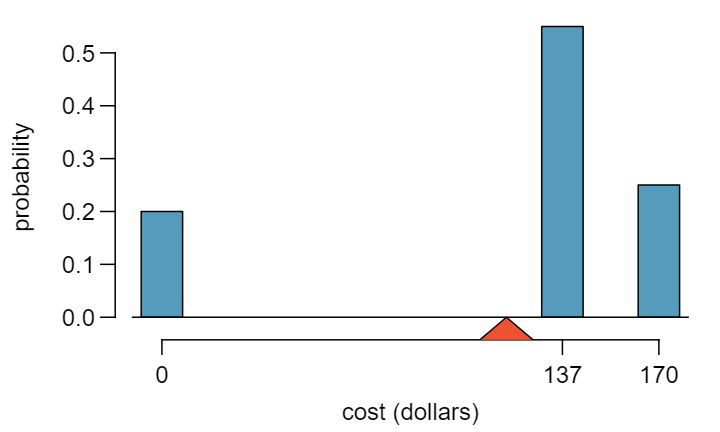What is the average revenue per student for this course?

Solution

The expected total revenue is $11,785, and there are 100 students. Therefore the expected revenue per student is $11,785/100 = 117.85\text{.}$ ### Subsection3.5.1Probability distributions A probability distribution is a table of all disjoint outcomes and their associated probabilities. Table 3.5.8 shows the probability distribution for the sum of two dice. ###### Rules for probability distributions A probability distribution is a list of the possible outcomes with corresponding probabilities that satisfies three rules: 1. The outcomes listed must be disjoint. 2. Each probability must be between 0 and 1. 3. The probabilities must total 1. Table 3.5.9 suggests three distributions for household income in the United States. Only one is correct. Which one must it be? What is wrong with the other two? 2 The probabilities of (a) do not sum to 1. The second probability in (b) is negative. This leaves (c), which sure enough satisfies the requirements of a distribution. One of the three was said to be the actual distribution of US household incomes, so it must be (c). Chapter 2 emphasized the importance of plotting data to provide quick summaries. Probability distributions can also be summarized in a histogram or bar plot. The probability distribution for the sum of two dice is shown in Table 3.5.8 and its histogram is plotted in Figure 3.5.10. The distribution of US household incomes is shown in Figure 3.5.11 as a bar plot. The presence of the 100+ category makes it difficult to represent it with a regular histogram. 3 It is also possible to construct a distribution plot when income is not artificially binned into four groups. Density histograms for continuous distributions are considered in Section 3.6.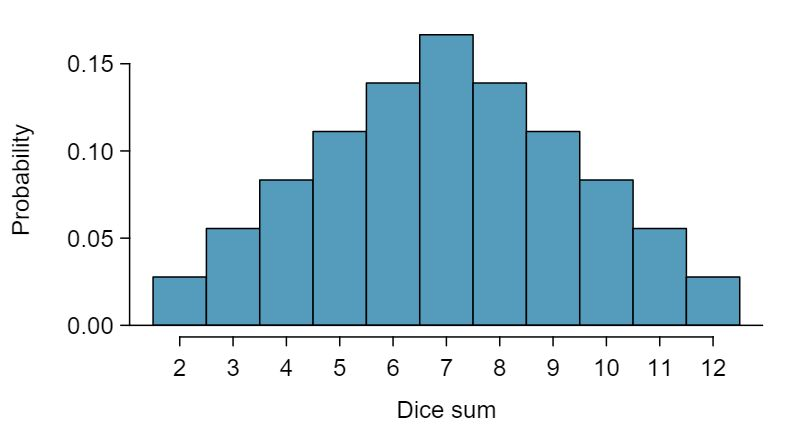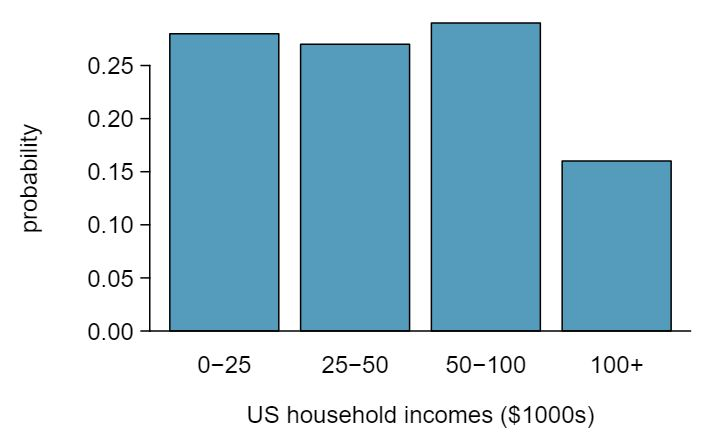In these bar plots, the bar heights represent the probabilities of outcomes. If the outcomes are numerical and discrete, it is usually (visually) convenient to make a histogram, as in the case of the sum of two dice. Another example of plotting the bars at their respective locations is shown in Figure 3.5.5. ### Subsection3.5.2Expectation We call a variable or process with a numerical outcome a random variable, and we usually represent this random variable with a capital letter such as $X\text{,}$ $Y\text{,}$ or $Z\text{.}$ The amount of money a single student will spend on her statistics books is a random variable, and we represent it by $X\text{.}$ ###### Random variable A random process or variable with a numerical outcome. The possible outcomes of $X$ are labeled with a corresponding lower case letter $x$ and subscripts. For example, we write $x_1=0\text{,}$ $x_2=137\text{,}$ and $x_3=170\text{,}$ which occur with probabilities $0.20\text{,}$ $0.55\text{,}$ and $0.25\text{.}$ The distribution of $X$ is summarized in Figure 3.5.5 and Table 3.5.12. We computed the average outcome of $X$ as$117.85 in Example 3.5.6. We call this average the expected value of $X\text{,}$ denoted by $E(X)$ The expected value of a random variable is computed by adding each outcome weighted by its probability:

\begin{align*} E(X) \amp = 0 \times P(X=0) + 137 \times P(X=137) + 170 \times P(X=170)\\ \amp = 0 \times 0.20 + 137 \times 0.55 + 170 \times 0.25 = 117.85 \end{align*}
###### Expected value of a discrete random variable

If $X$ takes outcomes $x_1\text{,}$ $x_2\text{,}$ ..., $x_n$ with probabilities $p_1\text{,}$ $p_2\text{,}$ ..., $p_n\text{,}$ the expected value of $X$ is the sum of each outcome multiplied by its corresponding probability:

\begin{align*} E(X) = \mu_x \amp = x_1\times p_1 + x_2\times p_2 + \cdots + x_n\times p_n \end{align*}
\begin{align} \amp = \sum_{i=1}^{n}(x_i\times p_i)\label{expected_value_equation}\tag{3.5.1} \end{align}

The expected value for a random variable represents the average outcome. For example, $E(X)=117.85$ represents the average amount the bookstore expects to make from a single student, which we could also write as $\mu=117.85\text{.}$ While the bookstore will make more than this on some students and less than this on other students, the average of many randomly selected students will be near 117.85. It is also possible to compute the expected value of a continuous random variable (see Section 3.6). However, it requires a little calculus and we save it for a later class. 4 $\mu_x = \int xf(x)dx$ where $f(x)$ represents a function for the density curve. In physics, the expectation holds the same meaning as the center of gravity. The distribution can be represented by a series of weights at each outcome, and the mean represents the balancing point. This is represented in Figure 3.5.5 and Figure 3.5.13. The idea of a center of gravity also expands to continuous probability distributions. Figure 3.5.14 shows a continuous probability distribution balanced atop a wedge placed at the mean.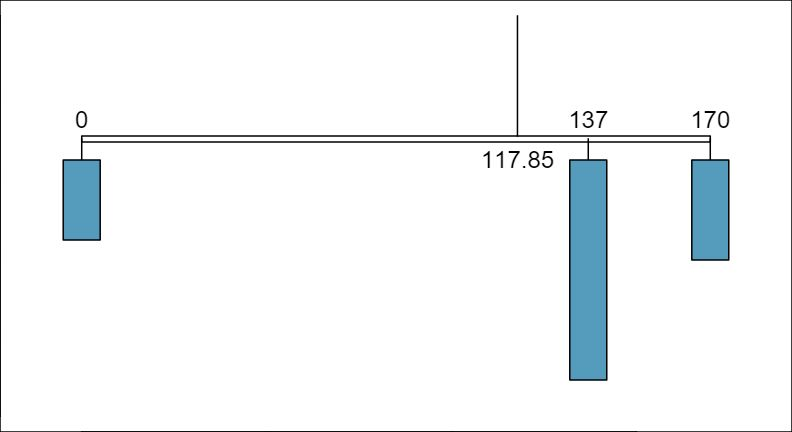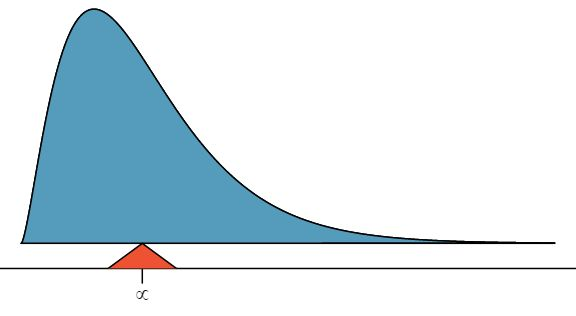### Subsection3.5.3Variability in random variables Suppose you ran the university bookstore. Besides how much revenue you expect to generate, you might also want to know the volatility (variability) in your revenue. The variance and standard deviation can be used to describe the variability of a random variable. Subsection 2.2.2 introduced a method for finding the variance and standard deviation for a data set. We first computed deviations from the mean ($x_i - \mu$), squared those deviations, and took an average to get the variance. In the case of a random variable, we again compute squared deviations. However, we take their sum weighted by their corresponding probabilities, just like we did for the expectation. This weighted sum of squared deviations equals the variance, and we calculate the standard deviation by taking the square root of the variance, just as we did in Subsection 2.2.2. ###### Variance and standard deviation of a discrete random variable If $X$ takes outcomes $x_1\text{,}$ $x_2\text{,}$ ..., $x_n$ with probabilities $p_1\text{,}$ $p_2\text{,}$ ..., $p_n$ and expected value $\mu_x=E(X)\text{,}$ then to find the standard deviation of $X\text{,}$ we first find the variance and then take its square root. \begin{align*} Var(X) = \sigma^2_x \amp = (x_1-\mu_x)^2\times p_1 + (x_2-\mu_x)^2\times p_2 + \cdots + (x_n-\mu_x)^2\times p_n\\ \amp = \sum_{i=1}^{n} (x_i - \mu_x)^2 \times p_i \end{align*} \begin{align} SD(X) = \sigma_x \amp = \sqrt{ \sum_{i=1}^{n} (x_i - \mu_x)^2 \times p_i}\label{random_variable_sd}\tag{3.5.2} \end{align} Just as it is possible to compute the mean of a continuous random variable using calculus, we can also use calculus to compute the variance. 5 $\sigma^2_x = \int (x - \mu_x)^2f(x)dx$ where $f(x)$ represents a function for the density curve. However, this topic is beyond the scope of the AP exam. Compute the expected value, variance, and standard deviation of $X\text{,}$ the revenue of a single statistics student for the bookstore. Solution It is useful to construct a table that holds computations for each outcome separately, then add up the results.  $i$ 1 2 3 Total $x_i$0 $137$170 $p_i$ 0.20 0.55 0.25 $x_i \times p_i$ 0 75.35 42.50 117.85

Thus, the expected value is $\mu=117.85\text{,}$ which we computed earlier. The variance can be constructed by extending this table:

 $i$ 1 2 3 Total $x_i$ $0$137 $170 $p_i$ 0.20 0.55 0.25 $x_i \times p_i$ 0 75.35 42.50 117.85 $x_i - \mu_X$ -117.85 19.15 52.15 $(x_i-\mu_X)^2$ 13888.62 366.72 2719.62 $(x_i-\mu_X)^2\times p_i$ 2777.7 201.7 679.9 3659.3 The variance of $X$ is $\sigma_x^2 = 3659.3\text{,}$ which means the standard deviation is $\sigma_x = \sqrt{3659.3} = 60.49\text{.}$ The bookstore also offers a chemistry textbook for$159 and a book supplement for $41. From past experience, they know about 25% of chemistry students just buy the textbook while 60% buy both the textbook and supplement. 6 (a) 100% - 25% - 60% = 15% of students do not buy any books for the class. Part (b) is represented by the first two lines in the table below. The expectation for part (c) is given as the total on the line $y_i\times p_i\text{.}$ The result of part (d) is the square-root of the variance listed on in the total on the last line: $\sigma_{\mbox{\tiny\itshape Y} } = \sqrt{Var(Y)} = 69.28\text{.}$  $i$ (scenario) 1 (noBook) 2 (textbook) 3 (both) Total $y_i$ 0.00 159.00 200.00 $p_i$ 0.15 0.25 0.60 $y_i\times p_i$ 0.00 39.75 120.00 $E(Y) = 159.75$ $y_i-\mu_Y$ -159.75 -0.75 40.25 $(y_i-\mu_Y)^2$ 25520.06 0.56 1620.06 $(y_i-\mu_Y)^2\times p_i$ 3828.0 0.1 972.0 $Var(Y) \approx 4800$ 1. What proportion of students don't buy either book? Assume no students buy the supplement without the textbook. 2. Let $Y$ represent the revenue from a single student. Write out the probability distribution of $Y\text{,}$ i.e. a table for each outcome and its associated probability. 3. Compute the expected revenue from a single chemistry student. 4. Find the standard deviation to describe the variability associated with the revenue from a single student. ### Subsection3.5.4Linear transformations of a random variable Let X be a random variable that represents how many books per student a textbook company sells. The probability distribution of X is given in the following table.  $x_i$ 1 2 3 $p_i$ 0.6 0.3 0.1 Using the methods of the previous section we can find that the mean $\mu_x = 1.5$ and the standard deviation $\sigma_x = 0.67\text{.}$ Suppose that the revenue the textbook company makes per student is$150 and that each book has a fixed cost of $30. The profit function, then, is $150X - 30\text{,}$ where X is the number of books sold. To calculate the mean and standard deviation for the profit of the textbook company, we could define a new variable $Y$ as follows: \begin{gather*} Y = 150X - 30 \end{gather*} Verify that the distribution of $Y$ is given by the table below. 7 $150 \times 1 - 30 = 120\text{;}$ $150 \times 2 - 30 = 270\text{;}$ $150 \times 3 - 30 = 420$  $y_i$$120 $270$420 $p_i$ 0.6 0.3 0.1

Using this new table, we can compute the mean and standard deviation of the textbook company's profit. However, because Y is a linear transformation of X, we can use the properties from Subsection 2.2.6. Recall that multiplying every X by 150 multiplies both the mean and standard deviation by 150. Subtracting 30 only subtracts 30 from the mean, not the standard deviation. Therefore,

\begin{align*} \mu_{150X-30}\amp =E(150X-30) \amp \sigma_{150X-30}\amp =SD(150X-30)\\ \amp = 150\times E(X) - 30 \amp \amp = 150\times SD(X)\\ \amp = 150\times 1.5 - 30 \amp \amp = 150\times 0.67\\ \amp = 195 \amp \amp = 100.5 \end{align*}

For a randomly selected student, the textbook company can expect to make $195 dollars, with a standard deviation of$100.50.

###### Linear transformations of a random variable

If $X$ is a random variable, then a linear transformation is given by $aX + b\text{,}$ where $a$ and $b$ are some fixed numbers.

\begin{align*} E(aX+b) \amp = a\times E(X) + b \amp SD(aX+b) \amp = \lvert a\rvert \times SD(X) \end{align*}

### Subsection3.5.5Linear combinations of random variables

So far, we have thought of each variable as being a complete story in and of itself. Sometimes it is more appropriate to use a combination of variables. For instance, the amount of time a person spends commuting to work each week can be broken down into several daily commutes. Similarly, the total gain or loss in a stock portfolio is the sum of the gains and losses in its components.

John travels to work five days a week. We will use $X_1$ to represent his travel time on Monday, $X_2$ to represent his travel time on Tuesday, and so on. Write an equation using $X_1\text{,}$ ..., $X_5$ that represents his travel time for the week, denoted by $W\text{.}$

Solution

His total weekly travel time is the sum of the five daily values:

\begin{equation*} W = X_1 + X_2 + X_3 + X_4 + X_5 \end{equation*}

Breaking the weekly travel time $W$ into pieces provides a framework for understanding each source of randomness and is useful for modeling $W\text{.}$

It takes John an average of 18 minutes each day to commute to work. What would you expect his average commute time to be for the week?

Solution

We were told that the average (i.e. expected value) of the commute time is 18 minutes per day: $E(X_i) = 18\text{.}$ To get the expected time for the sum of the five days, we can add up the expected time for each individual day:

\begin{align*} E(W) \amp = E(X_1 + X_2 + X_3 + X_4 + X_5)\\ \amp = E(X_1) + E(X_2) + E(X_3) + E(X_4) + E(X_5)\\ \amp = 18 + 18 + 18 + 18 + 18 = 90\text{ minutes } \end{align*}

The expectation of the total time is equal to the sum of the expected individual times. More generally, the expectation of a sum of random variables is always the sum of the expectation for each random variable.

Elena is selling a TV at a cash auction and also intends to buy a toaster oven in the auction. If $X$ represents the profit for selling the TV and $Y$ represents the cost of the toaster oven, write an equation that represents the net change in Elena's cash. 8 She will make $X$ dollars on the TV but spend $Y$ dollars on the toaster oven: $X-Y\text{.}$

Based on past auctions, Elena figures she should expect to make about $175 on the TV and pay about$23 for the toaster oven. In total, how much should she expect to make or spend?  9 $E(X-Y) = E(X) - E(Y) = 175 - 23 = 152\text{.}$ She should expect to make about $152. Would you be surprised if John's weekly commute wasn't exactly 90 minutes or if Elena didn't make exactly$152? Explain. 10 No, since there is probably some variability. For example, the traffic will vary from one day to next, and auction prices will vary depending on the quality of the merchandise and the interest of the attendees.

Two important concepts concerning combinations of random variables have so far been introduced. First, a final value can sometimes be described as the sum of its parts in an equation. Second, intuition suggests that putting the individual average values into this equation gives the average value we would expect in total. This second point needs clarification — it is guaranteed to be true in what are called linear combinations of random variables.

A linear combination of two random variables $X$ and $Y$ is a fancy phrase to describe a combination

\begin{equation*} aX + bY \end{equation*}

where $a$ and $b$ are some fixed and known numbers. For John's commute time, there were five random variables — one for each work day — and each random variable could be written as having a fixed coefficient of 1:

\begin{equation*} 1X_1 + 1 X_2 + 1 X_3 + 1 X_4 + 1 X_5 \end{equation*}

For Elena's net gain or loss, the $X$ random variable had a coefficient of +1 and the $Y$ random variable had a coefficient of -1.

When considering the average of a linear combination of random variables, it is safe to plug in the mean of each random variable and then compute the final result. For a few examples of nonlinear combinations of random variables — cases where we cannot simply plug in the means — see the footnote. 11 If $X$ and $Y$ are random variables, consider the following combinations: $X^{1+Y}\text{,}$ $X\times Y\text{,}$ $X/Y\text{.}$ In such cases, plugging in the average value for each random variable and computing the result will not generally lead to an accurate average value for the end result.

###### Linear combinations of random variables and the average result

If $X$ and $Y$ are random variables, then a linear combination of the random variables is given by $aX + bY\text{,}$ where $a$ and $b$ are some fixed numbers. To compute the average value of a linear combination of random variables, plug in the average of each individual random variable and compute the result:

\begin{gather*} E(aX+bY) = a\times E(X) + b\times E(Y) \end{gather*}

Recall that the expected value is the same as the mean, i.e. $E(X) = \mu_X\text{.}$

Leonard has invested $6000 in Google Inc. (stock ticker: GOOG) and$2000 in Exxon Mobil Corp. (XOM). If $X$ represents the change in Google's stock next month and $Y$ represents the change in Exxon Mobil stock next month, write an equation that describes how much money will be made or lost in Leonard's stocks for the month.

Solution

For simplicity, we will suppose $X$ and $Y$ are not in percents but are in decimal form (e.g. if Google's stock increases 1%, then $X=0.01\text{;}$ or if it loses 1%, then $X=-0.01$). Then we can write an equation for Leonard's gain as

\begin{gather*} 6000\times X + 2000\times Y \end{gather*}

If we plug in the change in the stock value for $X$ and $Y\text{,}$ this equation gives the change in value of Leonard's stock portfolio for the month. A positive value represents a gain, and a negative value represents a loss.

Suppose Google and Exxon Mobil stocks have recently been rising 2.1% and 0.4% per month, respectively. Compute the expected change in Leonard's stock portfolio for next month. 12 $E(6000\times X + 2000\times Y) = 6000\times 0.021 + 2000\times 0.004 = 134\text{.}$

You should have found that Leonard expects a positive gain in Guided Practice 3.5.24. However, would you be surprised if he actually had a loss this month? 13 No. While stocks tend to rise over time, they are often volatile in the short term.

### Subsection3.5.6Variability in linear combinations of random variables

###### Standard deviation of linear combinations of random variables

To find the standard deviation of a linear combination of random variables, we first consider $aX$ and $bY$ separately. We find the standard deviation of each, and then we apply the equation for the standard deviation of the sum of two variables:

\begin{gather*} SD_{aX + bY} = \sqrt{(a\times SD_X)^2 + (b\times SD_Y)^2} \end{gather*}

This equation is valid as long as the random variables $X$ and $Y$ are independent of each other.

Suppose John's daily commute has a standard deviation of 4 minutes. What is the uncertainty in his total commute time for the week?

Solution

The expression for John's commute time is

\begin{gather*} X_1 + X_2 + X_3 + X_4 + X_5 \end{gather*}

Each coefficient is 1, so the standard deviation of the total weekly commute time is

\begin{align*} \text{ SD } \amp = \sqrt{(1 \times 4)^2 + (1 \times 4)^2 + (1 \times 4)^2 + (1 \times 4)^2 + (1 \times 4)^2}\\ \amp = \sqrt{5\times (4)^2}\\ \amp = 8.94 \end{align*}

The standard deviation for John's weekly work commute time is about 9 minutes.

The computation in Example 3.5.28 relied on an important assumption: the commute time for each day is independent of the time on other days of that week. Do you think this is valid? Explain. 15 One concern is whether traffic patterns tend to have a weekly cycle (e.g. Fridays may be worse than other days). If that is the case, and John drives, then the assumption is probably not reasonable. However, if John walks to work, then his commute is probably not affected by any weekly traffic cycle.

Consider Elena's two auctions from Guided Practice 3.5.20. Suppose these auctions are approximately independent and the variability in auction prices associated with the TV and toaster oven can be described using standard deviations of $25 and$8. Compute the standard deviation of Elena's net gain. 16 The equation for Elena can be written as: $(1)\times X + (-1)\times Y\text{.}$ To find the SD of this new variable we do:

\begin{gather*} SD_{(1)\times X + (-1)\times Y} = \sqrt{(1\times SD_X)^2 + (-1\times SD_Y)^2 = (1\times 25)^2 + (-1\times 8)^2} = 26.25 \end{gather*}
Consider again Guided Practice 3.5.30. The negative coefficient for $Y$ in the linear combination was eliminated when we squared the coefficients. This generally holds true: negatives in a linear combination will have no impact on the variability computed for a linear combination, but they do impact the expected value computations.